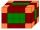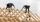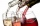# Cube, cuboid, and sphere

Volumes of a cube and a cuboid are in ratio 3: 2. Volumes of sphere and cuboid are in ratio 1: 3. In what ratio are the volumes of cube, cuboid, and sphere?

Result

x = (Correct answer is: 9:6:2)#### Solution:Leave us a comment of example and its solution (i.e. if it is still somewhat unclear...):

Showing 0 comments:Be the first to comment!#### To solve this example are needed these knowledge from mathematics:

Need help calculate sum, simplify or multiply fractions? Try our fraction calculator. Tip: Our volume units converter will help you with converion of volume units.

## Next similar examples:

1. CuboidFind the cuboid that has the same surface area as the volume.
2. Water levelThe glass container has a cuboid shape with dimensions of the bottom 24 cm and 12 cm. The height of water is 26 cm. Calculate the volume of the body, which are sunk into the water if the water level rise by 3 cm.
3. Inscribed sphereHow many % of the volume of the cube whose edge is 6 meters long is a volume of a sphere inscribed in that cube?
4. Cube cornersFrom cube of edge 14 cm cut off all vertices so that each cutting plane intersects the edges 1 cm from the nearest vertice. How many edges will have this body?
5. Cube cornersThe wooden cube with edge 64 cm was cut in 3 corners of cube with edge 4 cm. How many cubes of edge 4 cm can be even cut?
6. BallsPing pong balls have a diameter of approximately 4.6 cm. They are sold in boxes of 4 pieces: each box has the shape of a cuboid with a square base. The balls touch the walls of the box. Calculate what portion of the internal volume of the box is filled w
7. Volume of three cuboidsCalculate the total volume of all cuboids for which the the size of the edges are in a ratio of 1:2:3, and one of the edges has a size 6 cm.
8. CarpenterFrom wooden block carpenter cut off a small cuboid block with half the edge length. How many percent of wood he cut off?
9. Swimming poolThe pool shape of cuboid is 299 m3 full of water. Determine the dimensions of its bottom if water depth is 282 cm and one bottom dimension is 4.7 m greater than the second.
10. Cuboid - complicatefThree walls of the same cuboid has content 6 cm2, 10 cm2 and 15 cm2. Calculate the volume of the cuboid.
11. GasholderThe gasholder has spherical shape with a diameter 20 m. How many m3 can hold in?
12. Hemispherical hollowThe vessel hemispherical hollow is filled with water to a height of 10 cm =. How many liters of water are inside if the inside diameter of the hollow is d = 28cm?
13. Cross-sections of a coneCone with base radius 16 cm and height 11 cm divide by parallel planes to base into three bodies. The planes divide the height of the cone into three equal parts. Determine the volume ratio of the maximum and minimum of the resulting body.
14. WineryThe wine processing plant sold 8/15 of its total wine production to the domestic market, 2/7 to the foreign the rest of the wine. How many percents are still in stock?
15. Theorem proveWe want to prove the sentense: If the natural number n is divisible by six, then n is divisible by three. From what assumption we started?
16. TetrahedronCalculate height and volume of a regular tetrahedron whose edge has a length 18 cm.
17. BottlesThe must is sold in 5-liter and 2-liter bottles. Mr Kucera bought a total of 216 liters in 60 bottles. How many liters did Mr. Kucera buy in five-liter bottles?